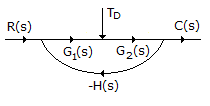# Electronics and Communication Engineering - Automatic Control Systems

Exercise : Automatic Control Systems - Section 4
1.
Examine the following statements
1. Air and gases are compressible.
2. Air, gases and some liquids are compressible.
3. Oil can function as hydraulic fluid as well as lubricator.
Which of the above statements are correct?
3 only
2 and 3 only
1 and 3 only
2 only
Explanation:
No answer description is available. Let's discuss.

2.
Which control action is also called rate control?
Proportional
Derivative
Integral
Proportional plus integral
Explanation:
No answer description is available. Let's discuss.

3.
The gain margin for a stable system
has a positive decibel value
has a negative decibel value
has a large negative decibel value
none of the above
Explanation:

For a stable system gain margin is positive. Therefore decibel value is also positive.

4.
The signal flow graph of a system is shown in the given figure. The effect of disturbance TD can be reduced byincreasing G2 (s)
decreasing G2 (s)
increasing G1 (s)
decreasing G1 (s)